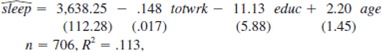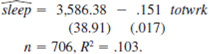Problem

# In Problem 3.3, we estimated the equationwhere we now report standard errors along with th...

In Problem 3.3, we estimated the equationwhere we now report standard errors along with the estimates.

(i) Is either educ or age individually significant at the 5% level against a two-sided alternative? Show your work.

(ii) Dropping educ and age from the equation givesAre educ and age jointly significant in the original equation at the 5% level? Justify your answer.

(iii) Does including educ and age in the model greatly affect the estimated tradeoff between sleeping and working?

(iv) Suppose that the sleep equation contains heteroskedasticity. What does this mean about the tests computed in parts (i) and (ii)?

#### Step-by-Step Solution

Solution 1

(i)

With $$d f=706-4=702$$, use the standard normal critical value ( $$d f=8$$ from the table), which is $$1.96$$ for a two-tailed test at the $$5 \%$$ level. The t-statistic is calculated as shown below:

\begin{aligned} t-\text { statistics } &=\frac{-11.13}{5.88} \\ & \approx-1.89 \end{aligned}

Since,

\begin{aligned} \left|t_{\text {efunc }}\right| &=1.89 \\ &<1.96 \end{aligned}

Hence, researcher fails to reject $$H_{0}: \beta_{\text {edwe }}=0$$ at the $$5 \%$$ level. Also, $$t$$-statistic for age is calculated below:

\begin{aligned} t-\text { statistics } &=\frac{2.20}{1.45} \\ &=1.52 \end{aligned}

Hence,

$$t_{\text {age }} \approx 1.52$$

So, age is also statistically insignificant at the $$5 \%$$ level.

(ii)

There is need to compute the $$R$$-squared form of the $$F$$ statistic for joint significance:

\begin{aligned} F-t e s t &=\left[\frac{.113-.103}{1-.113}\right](702 / 2) \\ & \approx 3.39 \end{aligned}

The $$5 \%$$ critical value in the $$F_{2,702}$$ distribution from the table with denominator $$d f=8: c v=3.00$$. Therefore, educ and age are jointly significant at the $$5 \%$$ level $$(3.39>3.00) .$$ In fact, the $$p$$-value is about $$0.019$$, and so educ and age are jointly significant at the $$2 \%$$ level.

(iii)

No, the variables educ and age in the model does not greatly affect the estimated tradeoff between sleeping and working. These variables are jointly significant, but including them only changes the coefficient on totwrk from $$-0.151$$ to $$-0.148$$.

(iv)

The standard t and F statistics that researcher used assume homoscedasticity, in addition to the other CLM assumptions. If there is heteroskedasticity in the equation, the tests are no longer valid and the results will be biased.